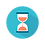From: Bayesian Models for Astrophysical Data, Cambridge Univ. Press

(c) 2017,  Joseph M. Hilbe, Rafael S. de Souza and Emille E. O. Ishida

you are kindly asked to include the complete citation if you used this material in a publication

Code 6.26 Create synthetic negative binomial data

========================================

require(R2jags)
require(MASS)

nobs <- 750

x1 <- runif(nobs)
xb <- 2 - 5 * x1

exb <- exp(xb)
theta <- 0.5
Q = 1.4

nbpy <- rnegbin(n=nobs, mu = exb, theta = theta*exb^Q)
TP <- data.frame(nbpy, x1)

========================================

Code 6.27 Bayesian three-parameter NB-P – indirect parameterization with zero trick

==================================================================

X <- model.matrix(~ x1 , data = TP)
K <- ncol(X)                                                                                  # number of betas

model.data <- list(Y = TP\$nbpy,                                                   # response
X = X,                                                              # covariates
N = nobs,                                                         # sample size
K =K)

sink("NBPreg.txt")

cat("
model{
# Diffuse normal priors on betas
for (i in 1:K) { beta[i] ~ dnorm(0, 0.0001) }

# Prior for dispersion
theta ~ dgamma(0.001,0.001)

# Uniform prior for Q
Q ~ dunif(0,3)

# NB-P likelihood using the zero trick
for (i in 1:N){
theta_eff[i]<- theta*(mu[i]^Q)
Y[i] ~ dnegbin(p[i], theta_eff[i])
p[i] <- theta_eff[i]/(theta_eff[i] + mu[i])
log(mu[i]) <- eta[i]
eta[i] <- inprod(beta[], X[i,])
}
}
"
,fill = TRUE)

sink()

# Inits function
inits <- function () {
list(beta = rnorm(K, 0, 0.1),
theta = 1,
Q =1)
}

# Parameters to display n output
params <- c("beta",
"theta",
"Q")

NBP <- jags(data = model.data,
inits = inits,
parameters = params,
model = "NBPreg.txt",
n.thin = 1,
n.chains = 3,
n.burnin = 2500,
n.iter = 5000)

print(NBP, intervals=c(0.025, 0.975), digits=3)

==================================================================Output on screen:

Inference for Bugs model at "NBPreg.txt", fit using jags,

3 chains, each with 5000 iterations (first 2500 discarded)

n.sims = 7500 iterations saved

mu.vect        sd.vect             2.5%           97.5%      Rhat       n.eff

Q                        1.245          0.093            1.068             1.431      1.001      3100

beta                2.060         0.048            1.962             2.151      1.001      6200

beta               -5.125         0.205           -5.525           -4.718      1.002      2100

theta                    0.529         0.062            0.413             0.659      1.001      4300

deviance       3512.175          2.903      3508.601      3519.508       1.004      1200

For each parameter, n.eff is a crude measure of effective sample size,

and Rhat is the potential scale reduction factor (at convergence, Rhat=1).

DIC info (using the rule, pD = var(deviance)/2)

pD = 4.2 and DIC = 3516.4

DIC is an estimate of expected predictive error (lower deviance is better).